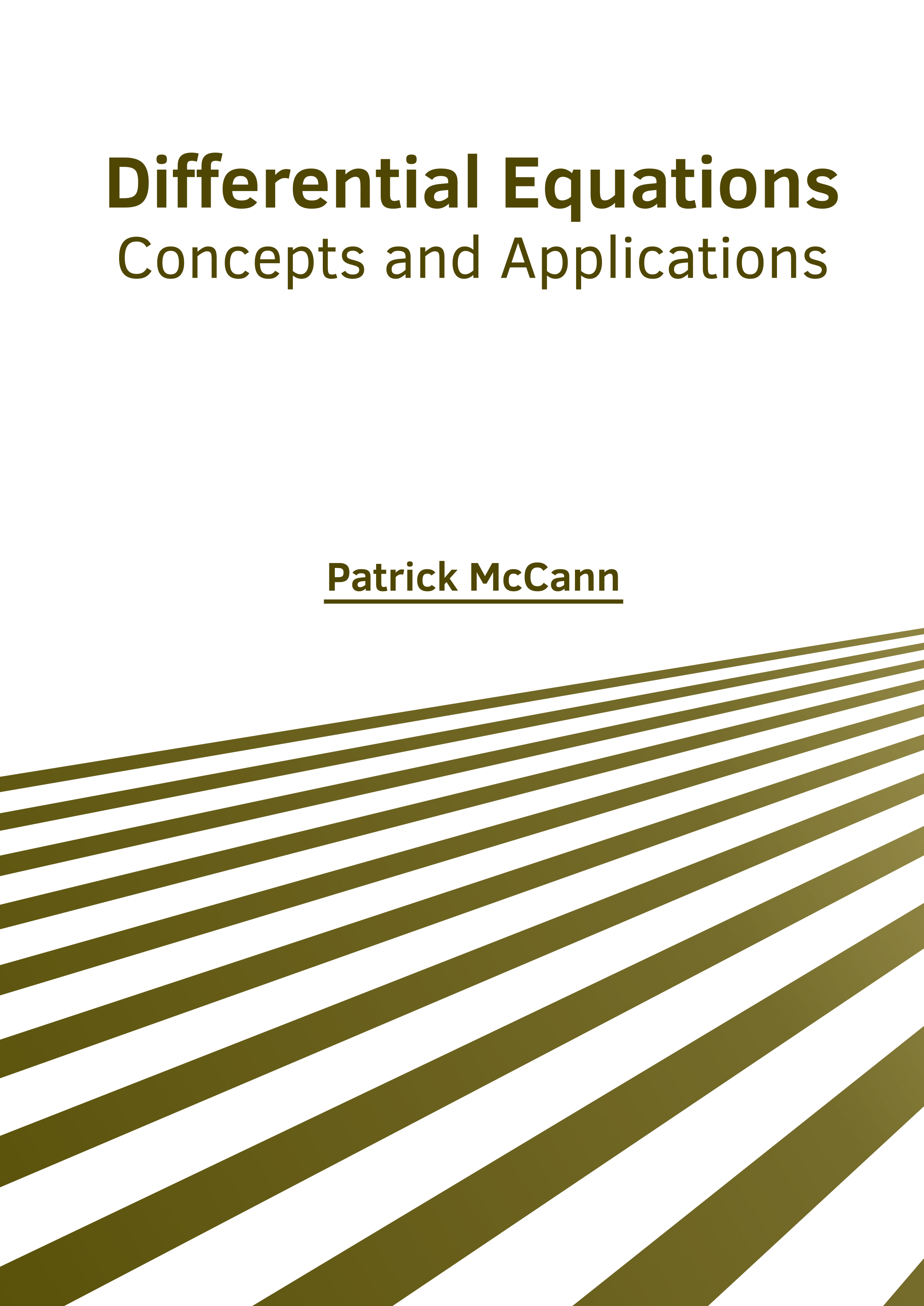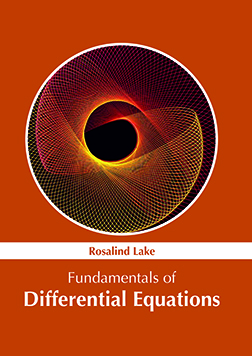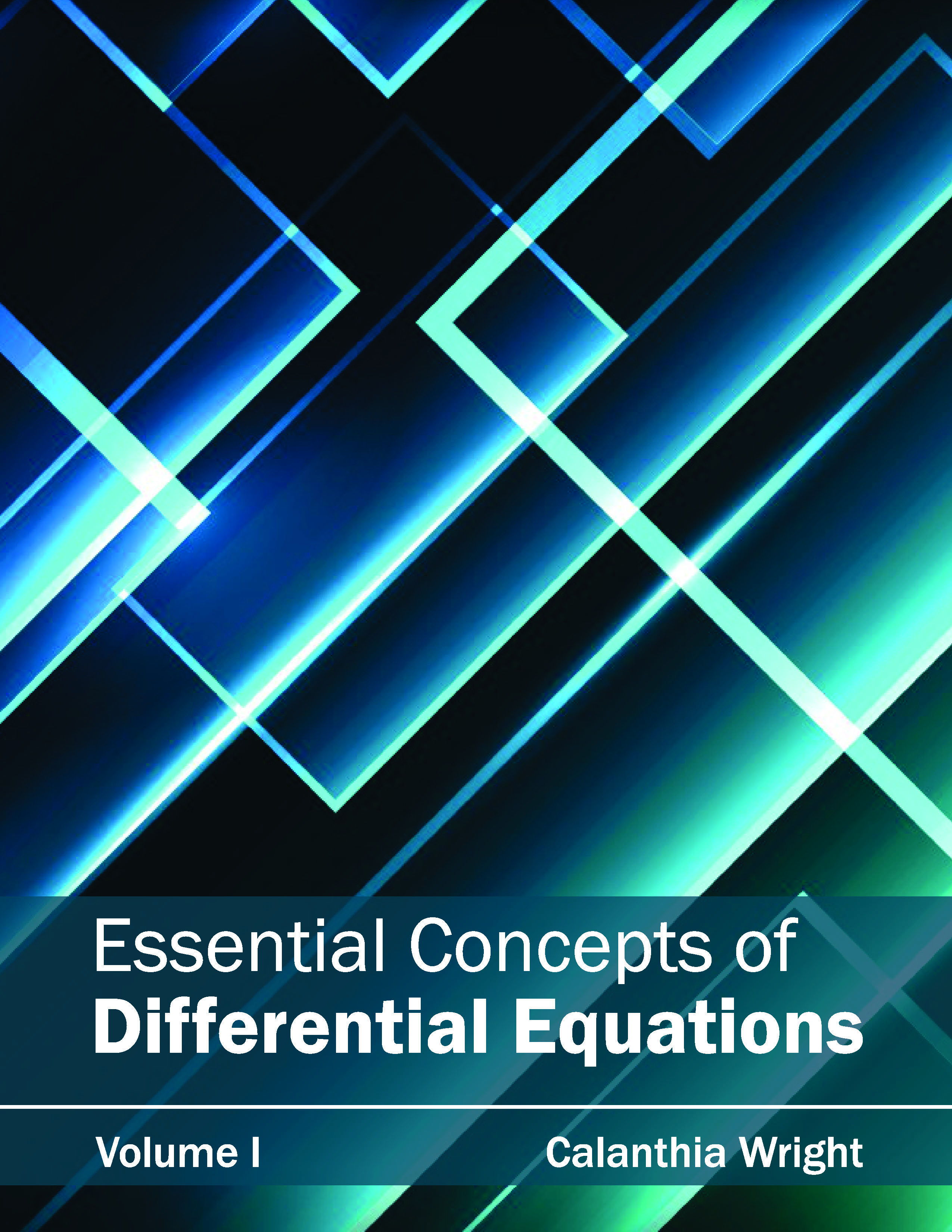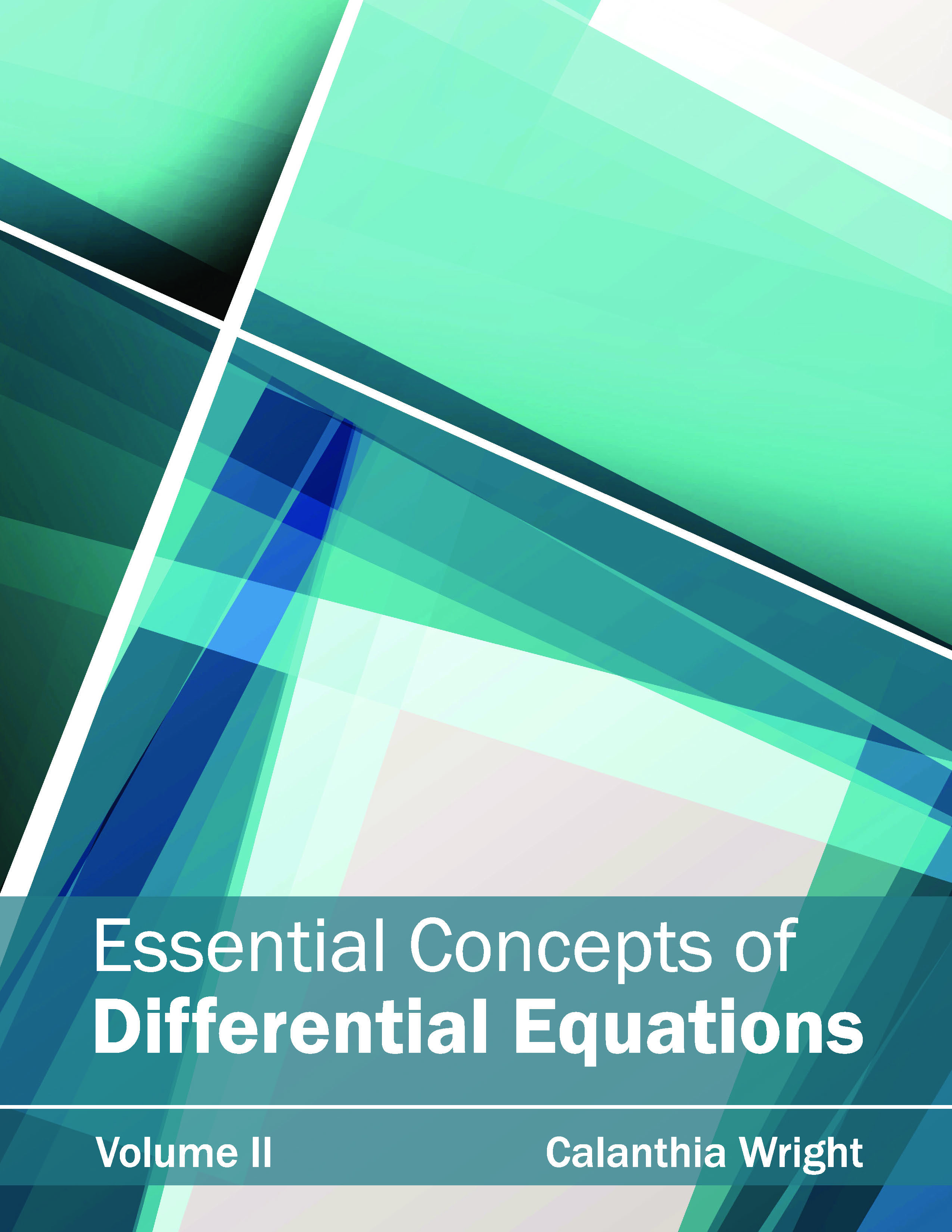BROWSE BY

#### Author

Results 1 - 4 of 4Differential Equations: Concepts and Applications Author : Patrick McCann Subject : Differential Equations ISBN :9781632387622 Differential Equation is a mathematical equation which denotes the relationship between a function and its derivatives. The functions usually represent physical quantities in the applications and the derivatives Read MoreFundamentals of Differential Equations Author : Rosalind Lake Subject : Differential Equations ISBN :9781632386021 The mathematical equations which define the relationship of a function with its derivatives are known as differential equations. The varied types of differential equations include ordinary, partial, non-linear Read MoreEssential Concepts of Differential Equations: Volume I Author : Calanthia Wright Subject : Differential Equations ISBN :9781632381828 This book attempts to understand the multiple branches and applications that fall under the field of differential equations and how such research can be useful in our lives. The various advancements that Read MoreEssential Concepts of Differential Equations: Volume II Author : Calanthia Wright Subject : Differential Equations ISBN :9781632381835 This book attempts to understand the multiple branches and applications that fall under the field of differential equations and how such research can be useful in our lives. The various advancements that Read More
Results 1 - 4 of 4